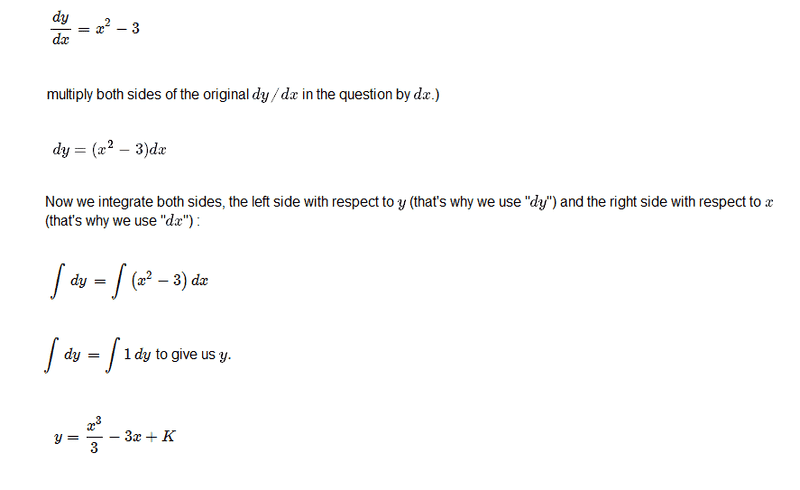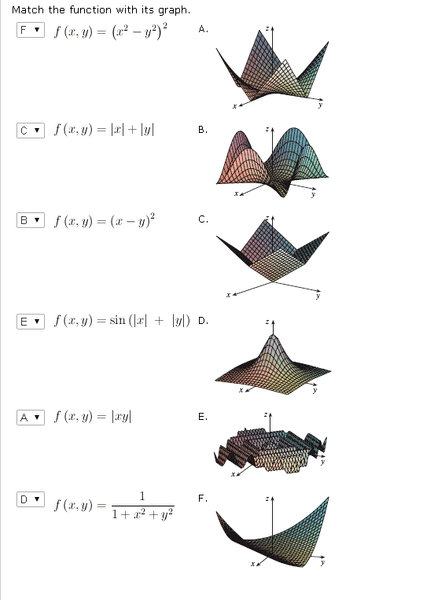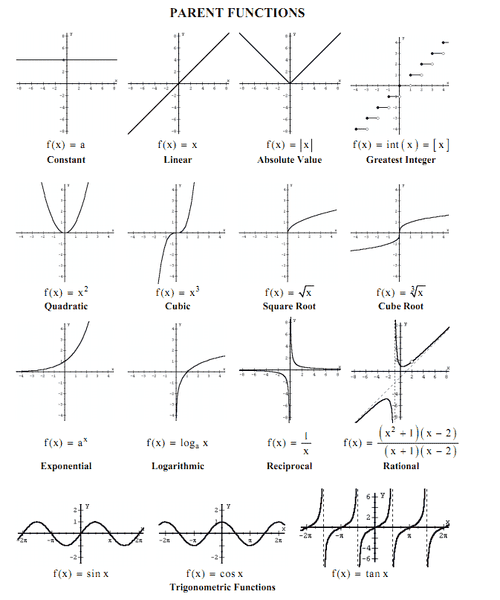# Few maths questions ..?

i have been trying to learn maths from some basics .. because i had to do programming related to maths which was in my college syllabus ...

i have been trying to narrow it down .. because we lacked proper texts and materials to learn it properly ...

the whole thing sort of looked a bit like this ...

mathematical expressions
equations in one variable
equations in two variables
system of 2 equations containing 2 variables
functions in one variable
functions in two variables

differential equations

first order differential equations
second order differential equations
higher order differential equations ...

linear differential equations
separable differential equations
exact differential equations
homogeneous differential equations
non homogeneous differential equations
using the method of undetermined coefficients ...

partial differential equations ...

Numerical Methods and errors
Interpolation
Numerical Differentiation
Numerical Integration
Solution of Algebraic and Transcendental Equations
Numerical Solution of a system of Linear Equations
Numerical Solution of Ordinary differential equations
Curve fitting
Numerical Solution of problems associated with Partial Differential Equations

Solution of Algebraic and Transcendental Equation
2.1 Introduction
2.2 Bisection Method
2.3 Method of false position
2.4 Iteration method
2.5 Newton-Raphson Method
2.6 Ramanujan's method
2.7 The Secant Method Finite Differences

3.1 Introduction
3.3.1 Forward differences
3.3.2 Backward differences
3.3.3 Central differences
3.3.4 Symbolic relations and separation of symbols
3.5 Differences of a polynomial Interpolation
3.6 Newton's formulae for intrapolation
3.7 Central difference interpolation formulae
3.7.1 Gauss' Central Difference Formulae
3.9 Interpolation with unevenly spaced points
3.9.1 Langrange's interpolation formula
3.10 Divided differences and their properties
3.10.1 Newton's General interpolation formula
3.11 Inverse interpolation Numerical Differentiation and Integration

5.1 Introduction
5.2 Numerical differentiation (using Newton's forward and backward formulae)
5.4 Numerical Integration
5.4.1 Trapizaoidal Rule
5.4.2 Simpson's 1/3-Rule
5.4.3 Simpson's 3/8-Rule Matrices and Linear Systems of equations
6.3 Solution of Linear Systems – Direct Methods
6.3.2 Gauss elimination
6.3.3 Gauss-Jordan Method
6.3.4 Modification of Gauss method to compute the inverse
6.3.6 LU Decomposition
6.3.7 LU Decomposition from Gauss elimination
6.4 Solution of Linear Systems – Iterative methods
6.5 The eigen value problem
6.5.1 Eigen values of Symmetric Tridiazonal matrix Numerical Solutions of Ordinary Differential Equations

7.1 Introduction
7.2 Solution by Taylor's series
7.3 Picard's method of successive approximations
7.4 Euler's method
7.4.2 Modified Euler's Method
7.5 Runge-Kutta method
7.6 Predictor-Corrector Methods
7.6.2 Milne's method

i was wondering about the difference between a differential equations and functions like f(x,y) =

??

NascentOxygen
Staff Emeritus
i was wondering about the difference between a differential equations and functions like f(x,y) =
Can we narrow this to "how would I recognize a differential equation if I were to encounter one"?

is it a differential equation ... ?? i am sorry i am new to differential equation too ...

so its a bit like, function in one variable vs a function in two variables...

i am not sure what to google to learn such functions ...

Nidum
Gold Member
Nidum ,

i have few more doubts ...
the simple differential equations i have encountered .. sorts of looked a bit like this ...

is this a function of one variable or two variables ... ??are these differential equations too ??

NascentOxygen
Staff Emeritus
is it a differential equation ... ?? i am sorry i am new to differential equation too ...

so its a bit like, function in one variable vs a function in two variables...

i am not sure what to google to learn such functions ...
Differential equations are different from the algebraic equations you've earlier encountered. If you google "differential equations" I'm sure you'll find everything you could hope for.

A differential equation might look like this: ##\color{blue}{\frac{dy}{dx}=x+3}##

##\frac{dy}{dx}## is a way of expressing the gradient of the curve, and this equation tells you the gradient of the curve as a function of x. There are an infinite number of curves whose gradients satisfy this equation, those curves being all identical except for their intercept on the y-axis. To specify exactly one of those curves, you just also need to indicate a point lying on it, any point will do.

You have already encountered differential equations, though they were in disguise. Recall this equation of motion: ##v=v_{\scriptsize0}+at##, and remember that acceleration is ##\frac{∆v}{∆t}##,
so the equation can be written as ##v=v_{\scriptsize0}+\frac{∆v}{∆t}t##,
or using differentials, as ##v=v_{\scriptsize 0}+\frac{dv}{dt}t##.

So differential equations are not totally new to you, even though this way of writing them is new.

thanks NascentOxygen ,

very nice explanation ...

the problem i was facing was that since i am not a native english speaker ...i don't always get the terminologies properly ... i might have to google terms like gradient and things like all that to understand what it really means in mathematics terms ...

few more doubts ..
i am basically trying to understand the difference between these two ..as for differential equations .. i have been making some notes for it in the last couple of days ..so i think i am a bit familiar with differential equations now ..

Last edited by a moderator:
NascentOxygen
Staff Emeritus